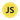# What’s the Difference Between Strict Equality vs. Loose Equality Operators in JavaScript?

Learn about the difference between strict equality vs. loose equality operators in JavaScript, and why it matters.

In JavaScript, you can use two equality operators to compare values, the loose equality operator `==` (double equals) and strict equality operator `===` (triple equals). They appear the same on the surface, but they are not, and it’s important to understand the difference because if you don’t, you can run into bugs.

Try running the following code in your console:

``````let a = 4 // number
let b = "4" // string

console.log(a == b) // output: true``````

Why does JavaScript say that the above is true when it’s not? We have a value of the type number being compared to a value of the type string — so how can they possibly be equal values?

That’s because when you use the loose equality operator `==` it converts the operands to the same type (if they are not already), and then compares their values.

This process called type coercion (when two values are compared after attempting to convert them into a common type).

So in the example above, the output of `true` is correct, because before the values are compared, their value types are converted to be the same.

Wow, that’s stupid!

I agree, but that’s how it is. What’s important is that you are aware of this inconvenient “quirk” of JavaScript.

And that’s where the strict equality operator `===` comes in and saves the day because it doesn’t convert the value type, it strictly compares value types and then checks for equality:

``````let a = 4
let b = "4"

console.log(a === b)
// output: false``````

Now we get `false`, because although both variables (a & b) contain the value “4”, the operands are different value types (a is a number value, b is a string value).

Unlike the non-strict `==` operator, the strict `===` operator gives you predictable outputs, which is why most developers advise against using `==`.

## Is there ever a good reason to use the non-strict comparison operator in JavaScript?

Rarely, but I’m sure you can find some examples. As a rule of thumb, default to using the strict `===` equality operator, unless there’s an obvious reason to use the non-strict `==` equality operator. This way you can rule out bugs in your code being caused by using the wrong equality operator, and that’s always a bonus.